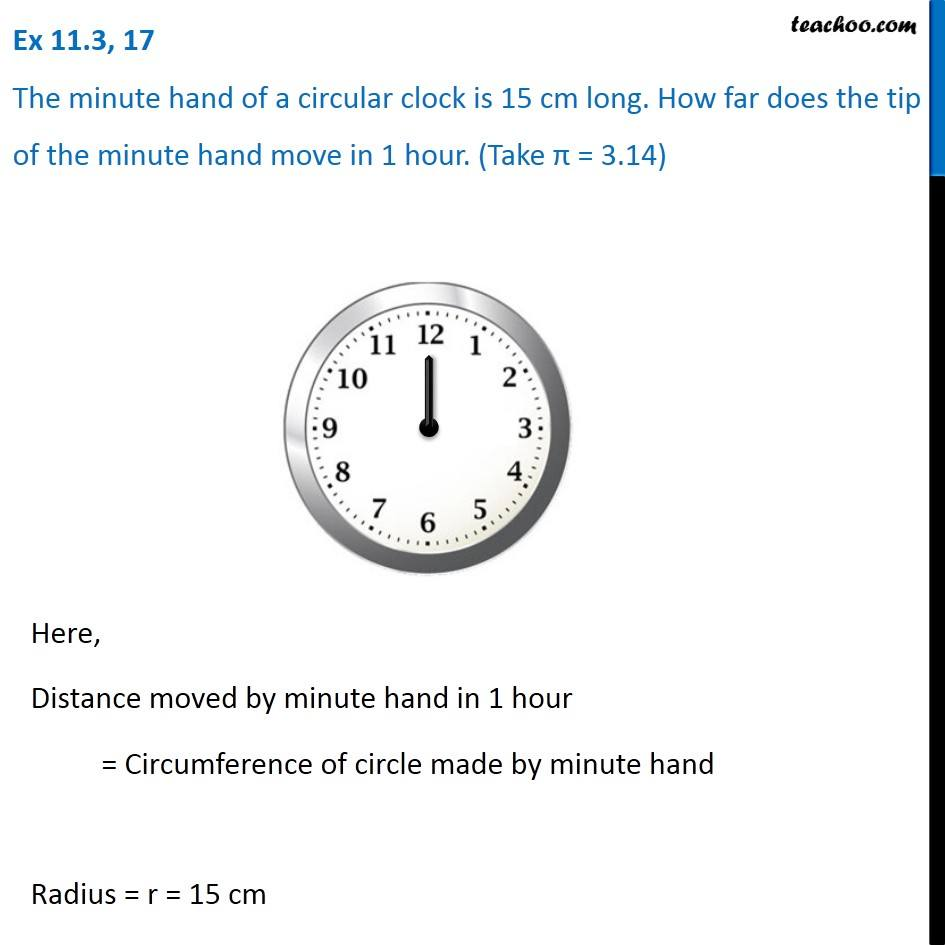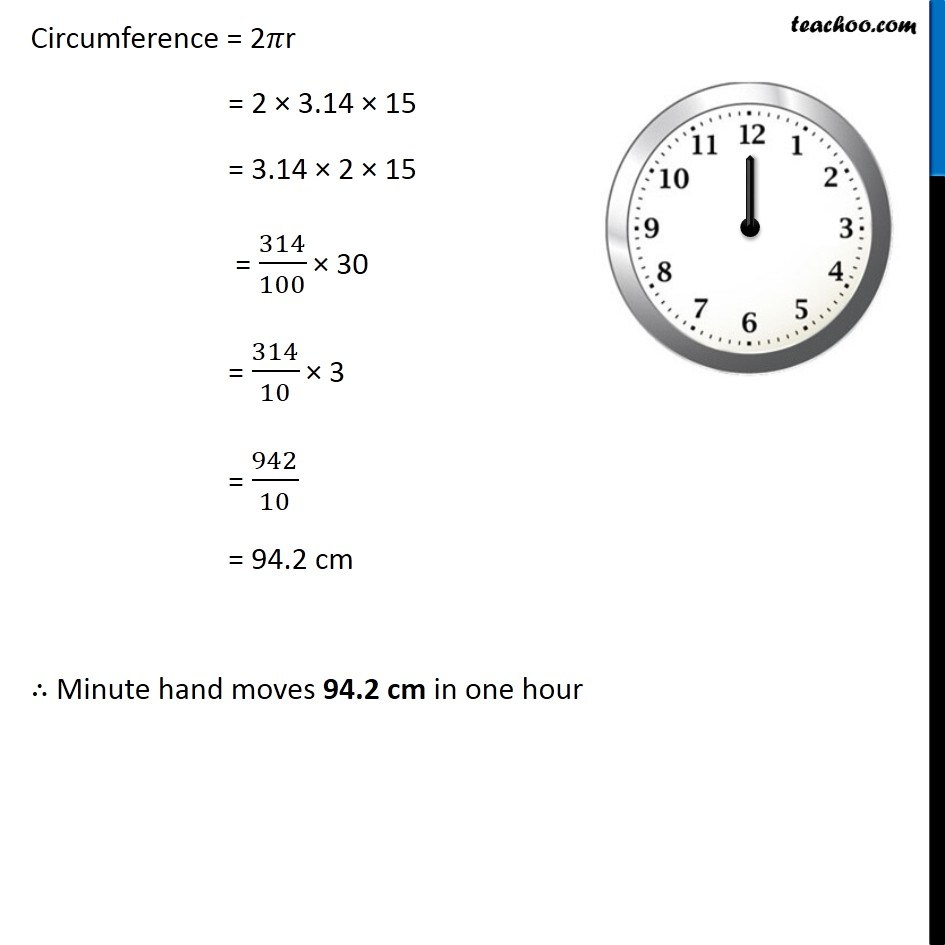1. Chapter 11 Class 7 Perimeter and Area
2. Concept wise
3. Circumference of circle

Transcript

Ex 11.3, 17 The minute hand of a circular clock is 15 cm long. How far does the tip of the minute hand move in 1 hour. (Take π = 3.14) Here, Distance moved by minute hand in 1 hour = Circumference of circle made by minute hand Radius = r = 15 cm Circumference = 2𝜋r = 2 × 3.14 × 15 = 3.14 × 2 × 15 = 314/100 × 30 = 314/10 × 3 = 942/10 = 94.2 cm ∴ Minute hand moves 94.2 cm in one hour